Courses

# Part Test - 16 (JEE Advanced 2021)

## 60 Questions MCQ Test Mock Test Series for JEE Main & Advanced 2022 | Part Test - 16 (JEE Advanced 2021)

Description
This mock test of Part Test - 16 (JEE Advanced 2021) for JEE helps you for every JEE entrance exam. This contains 60 Multiple Choice Questions for JEE Part Test - 16 (JEE Advanced 2021) (mcq) to study with solutions a complete question bank. The solved questions answers in this Part Test - 16 (JEE Advanced 2021) quiz give you a good mix of easy questions and tough questions. JEE students definitely take this Part Test - 16 (JEE Advanced 2021) exercise for a better result in the exam. You can find other Part Test - 16 (JEE Advanced 2021) extra questions, long questions & short questions for JEE on EduRev as well by searching above.
QUESTION: 1

Solution:
QUESTION: 2

### A planoconvex lens has a maximum thickness of 6 cm. When placed on a horizontal table with the curved surface in contact with the table surface, the apparent depth of the bottommost point of the lens is found to be 4 cm. If the lens is inverted such that the plane face of the lens is in contact with the surface of the table, the apparent depth of the center of the plane face is found to be (17/4) cm. The radius of curvature of the lens is ______.

Solution:
*Multiple options can be correct
QUESTION: 3

### Displacement (y) of air column of position x from its mean position at any instant is given by graph shown in the figure. We can conclude from graph that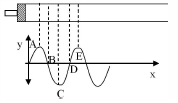Solution:

AB

Density of air is maximum at B

Density of air is minimum at D

*Multiple options can be correct
QUESTION: 4

A ring of radius R with four metalic wire connected as shown is rolling without sliding on a horizontal surface with a velocity of centre of mass v and angular velocity ω, in a uniform magnetic field B which is perpendicular to the plane of disc as shown in figure. O is the centre of disc and P, Q, R & S are the four points on disc.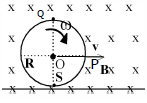Solution: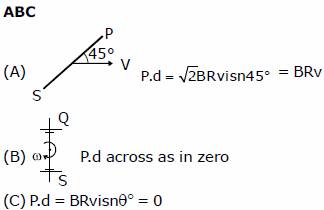QUESTION: 5

A prism of a certain angle deviates the red and blue rays by 8°and 12° respectively. Another prism of the same angle deviates the red and blue rays by 10° and 14° respectively. The prisms are small angled and made of different materials. The dispersive powers of the materials of the prisms are in the ratio

Solution:
QUESTION: 6

A radio active substance contains 10,000 nuclei and its half life period is 20 days. The number of nuclei present at the end of 10 days is

Solution:
QUESTION: 7

A ball rests upon a flat piece of paper on a table top. The paper is pulled horizontally but quickly towards right as shown. Relative to its initial position with respect to the table, the ball _____.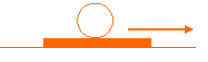(a) remains stationary if there is no friction between the paper and the ball.

(b) moves to the left and starts rolling backwards, i.e. to the left if there is a friction between the paper and the ball.

(c) moves forward, i.e. in the direction in which the paper is pulled.

Here, the correct statement/s is/are _____ .

Solution:
*Multiple options can be correct
QUESTION: 8

A body float on water and also on an oil of density 1.25. Which of the following is/are true ?

Solution: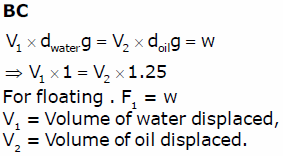QUESTION: 9

Two waves are represented by the equations yasin(wkx + 0.57)and y2 = acos(wkx)m, where is in meter and in s. The phase difference between them is

Solution:
QUESTION: 10

A missile is fired for maximum range with an initial velocity of 20 m/s. If g = 10 m /s2, the range of the missile is

Solution:
QUESTION: 11

In the circuit shown, both batteries are ideal. EMF E1 of battery 1 has a fixed value, but emf E2 of battery 2 can be varied between 1 V and 10 V. The graph gives the currents through the two batteries as a function of E2, but are not marked as which plot corresponds to which battery. But for both plots, current is assumed to be negative when the direction of the current through the battery is opposite the direction of that battery's emf. (direction from negative to positive)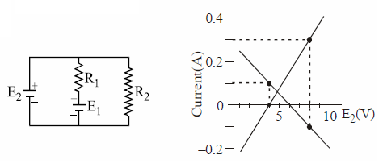Q. The value of emf E1 is

Solution: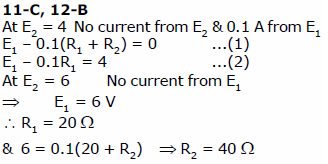QUESTION: 12

A and B are two infinitely long straight parallel conductors. C is another straight conductor of length 1 m kept parallel to A and B as shown in the figure. Then the force experienced by C is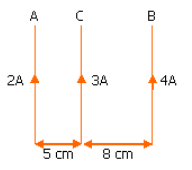Solution:
QUESTION: 13

A mirror is mounted on a stand as shown. The mirror has negligible mass. On the other side, massless arrow is mounted. The natural length of the spring is 1m. The whole system is on frictionless surface. The two bodies are pulled out such that spring is extended by 10 cm and released.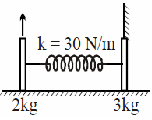Q. What is the minimum distance between arrow and its image during subsequent motion ?

Solution: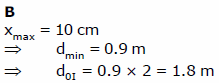QUESTION: 14

A mirror is mounted on a stand as shown. The mirror has negligible mass. On the other side, massless arrow is mounted. The natural length of the spring is 1m. The whole system is on frictionless surface. The two bodies are pulled out such that spring is extended by 10 cm and released.Q. What is the maximum velocity of the image of the arrow as seen by a person on the ground ?

Solution: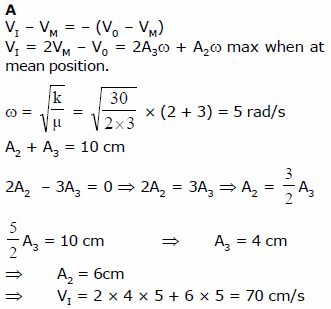QUESTION: 15

In the Wheatstone's network given below, P = 10 W, Q = 20W,  R = 15 W, S = 30W The current passing through the battery (of negligible internal resistance) is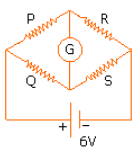Solution:
QUESTION: 16

The current passing through the ideal ammeter in the circuit given below is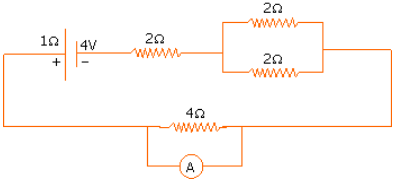Solution:
QUESTION: 17

Arrange the following electromagnetic radiations per quantum in the order of increasing energy:

A : Blue light
B : Yellow light
C : X-ray

Solution:
QUESTION: 18

Out of the following options which one can be used to produce a propagating electromagnetic wave?

Solution:
QUESTION: 19

The electric and magnetic field of an electromagnetic wave are

Solution:
QUESTION: 20

Vapour pressure of pure ‘A’ is 70 mm of Hg at 25 °C. It forms an ideal solution with ‘B’ in which mole fraction of A is 0.8. lf the vapour pressure of the solution is 84 mm of Hg at 25 °C, the vapour pressure of pure ‘B’ at 25 °C is

Solution:
*Multiple options can be correct
QUESTION: 21

Select correct statement(s)?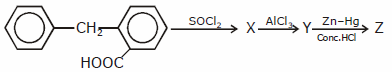Solution: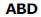are correct answers

*Multiple options can be correct
QUESTION: 22

Which of the following increase(s) the activation of a solid adsorbent ?

Solution: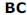are correct amswers

QUESTION: 23

When compared to DG0 for the formation of Al2O3 , the AG0 for the formation of Cr2O3 is

Solution:
*Multiple options can be correct
QUESTION: 24

Carbon monooxide is

Solution: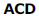are correct answers

*Multiple options can be correct
QUESTION: 25

Which of the following is/are endothermic reactions ?

Solution: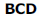are correct answers

*Multiple options can be correct
QUESTION: 26

Which of the following are correct order of basic strength ?

Solution: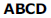are correct answers

*Multiple options can be correct
QUESTION: 27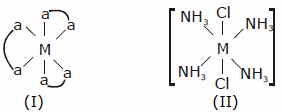Which of the following is correct related to I and II ?

Solution: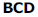are correct answers

QUESTION: 28

When conc. H2SO4 is heated with P2 05 , the acid is converted into

Solution:
QUESTION: 29

In Dumas’ method of estimation of nitrogen 0.35 g of an organic compound gave 55 mL of nitrogen collected at 300 K temperature and 715 mm pressure. The percentage composition of nitrogen in the compound would be

(Aqueous tension at 300 K = 15 mm)

Solution:
QUESTION: 30

In which one of the following, does the given amount of chlorine exert the least pressure in a vessel of capacity 1 dm3 at 273K?

Solution:

B

QUESTION: 31

Enthalpy of vapourization of benzene is +35.3 kJ mol1 at its boiling point of 80°C.

The entropy change in the transition of the vapour to liquid at its boiling point

[in JK1 mol1] is ______.

Solution:
QUESTION: 32

One mole of which of the following has the highest entropy?

Solution:
QUESTION: 33

Number of isomeric forms (constitutional and sterioisomers) for
[Rh(en)2(NO2)(SCN)]+ are

Solution:

D

QUESTION: 34

One gram of silver gets distributed between 10 cmof molten zinc and 100 cmof molten lead at 800°C. The percentage of silver still left in the lead layer is approximately

Solution:
QUESTION: 35

The activation energy of a reaction at a given temperature is found to be 2.303 RT J mol–1. The ratio of rate constant to the Arrhenius factor is

Solution:
QUESTION: 36

By X-ray studies, the packing of atoms in a crystal of gold is found to be in layers such that starting from any layer, every fourth layer is found to be exactly identical. The density of gold is found to be 19.4 g/cm3 and its atomic mass is 197 amu.

The length of the edge of the unit cell will be:

Solution: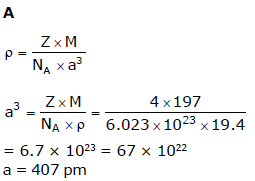QUESTION: 37

Which of the following elements is present as the impurity to the maximum extent in the pig iron?

Solution:
QUESTION: 38

Three moles of PCl5, three moles of PCIand two moles of Clz are taken in a closed vessel. If at equilibrium the vessel has 1.5 moles of PCl5, the number of moles of PCl3 present in it is

Solution:
QUESTION: 39

Vapour pressure of pure ‘A’ is 70 mm of Hg at 25 °C. It forms an ideal solution with ‘B’ in which mole fraction of A is 0.8. lf the vapour pressure of the solution is 84 mm of Hg at 25 °C, the vapour pressure of pure ‘B’ at 25 °C is

Solution:
QUESTION: 40

When compared to DG0 for the formation of Al2O3 , the AG0 for the formation of Cr2O3 is

Solution:
QUESTION: 41

When conc. H2SO4 is heated with P2 05 , the acid is converted into

Solution:
QUESTION: 42

The differentiation of sinx with respect to cosx is ?

Solution:
*Multiple options can be correct
QUESTION: 43

Let there are three points A (0, 4/3) B(–1, 0) and C(1, 0) in x – y plane. The distance from a variable point P to the line BC is the geometic mean of the distances from this point to lines AB and AC then locus of P can be

Solution:

B, D

QUESTION: 44

The number of disulphide linkages present in insulin are

Solution:
QUESTION: 45

The number of gram molecules of chlorine in 6.02 × 1025 hydrogen chloride molecules is

Solution:
QUESTION: 46

One mole of an organic compound ‘A’ with the formula C3H8O reacts completely with two moles of HI to form X and Y. When ‘Y’ is boiled with aqueous alkali forms Z.Z

answers the iodoform test. The compound ‘A’ is ______.

Solution:
QUESTION: 47

One dm3 solution containing 105 moles each of Cl ions and CrO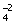ions is treated with 104 mole of silver nitrate. Which one of the following observations is made?

[KSP AgCrO4, = 4 X 1012]

[KSP AgCl = 1 x1010]

Solution:
QUESTION: 48

Decreasing order of reactivity of hydrogen halide acids in the conversion of ROH → RX is

Solution:
QUESTION: 49

The amount of heat evolved when 500 cm3 of 0.1 M HCI is mixed with 200 cm3of 0.2 M NaOH is ______.

Solution:
QUESTION: 50

Standard electrode potential for Sn4+ / Sn2+ couple is +0.15 V and that for the Cr3+ / Cr couple is –0.74 V. These two couples in their standard state are connected to make a cell. The cell potential will be

Solution: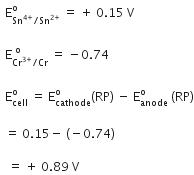QUESTION: 51

If one mole of ammonia and one mole of hydrogen chloride are mixed in a closed container to form ammonium chloride gas, then

Solution:
QUESTION: 52

If n = 6, the correct sequence of filling of electrons will be

Solution:
QUESTION: 53

The typical range of molar enthalpies for the strongest intermolecular (Hydrogen) bonds is

Solution:
QUESTION: 54

An organic compound on heating with CuO produces CO2 but no water. The organic compound may be

Solution:
QUESTION: 55

The normality of 30 volume H2O2 is

Solution:

B

QUESTION: 56

An element with atomic number 21 is a

Solution:
QUESTION: 57

Among the following, the compound that contains ionic, covalent and coordinate linkage is

Solution:
QUESTION: 58

A white crystalline salt A reacts with dilute HCI to liberate a suffocating gas B and also forms a yellow precipitate. The gas B turns potassium dichromate acidified with dilute H2SO4 to a green coloured solution C. A, B and C are respectively ______.

Solution:
QUESTION: 59

For a chemical reaction A → B, the rate of the reaction is 2 × 103 mol dm3 s1, when the initial concentration is 0.05 mol dm3. The rate of the same reaction is 1.6 × 102 mol dm3 s1when the initial concentration is 0.1 mol dm3. The order of the reaction is

Solution:
QUESTION: 60

Excess of carbon dioxide is passed through 50 ml of 0.5 M calcium hydroxide solution.  After the completion of the reaction, the solution was evaporated to dryness. The solid calcium carbonate was completely neutralised with 0.1 N Hydrochloric acid. The volume of Hydrochloric acid required is (At. mass of calcium = 40)

Solution: